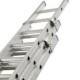# Brain Teaser: A Sliding LadderA 25 ft ladder (assume it is not an extension ladder) is placed with its foot 7 ft away from a building. If the top of the ladder slips down 4 ft, how many feet will the bottom slide out?

A distribution manager’s dilemma | Back – Next | Fill in the Blanks## Calculate the velocity of water coming out of a double-entry pipe

If we know the volumetric flow rates of the water going into the two branches, …

1.8ft isnt it??

2.25-7=18 ft. If your ladder slips 4 ft down the wall you are in trouble. My prediction is you are going to hit the ground.

3.It’s 8-FT. Looks like i’m a bit late witht hat answer but I thought I would join in anyway. Is this part of a math test or something then?

4.Thanks guys! 8 ft is correct.

5.assuming angle btwn wall and ground is 90 degrees, this makes it a right triangle problem.

sq rt((25**2) – (7**2)) = 24 (vert distance that ladder is up wall)
then, after the 4 ft “slip”,
24 – 4 = 20 is distance up wall (vert)

solve for new distance from wall (Horiz)
sq rt((25**2) – (20**2)) = 15

difference between old distance and new distance is what the “slip” was
15 – 7 = 8 ft

6.I get 8 ft. The initial vertical dimension is 24 ft.

If it slips 4 ft vertically, the new vertical dimension is 20 ft, making the new horizontal leg of 15 ft. Original horizontal was 7 ft, so it has slipped 8ft.